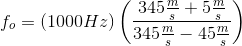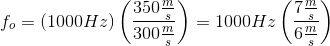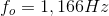# MCAT Physical : Doppler Effect

## Example Questions

### Example Question #1 : Doppler Effect

Which of the following best describes the effect of the Doppler shift on the appearance of stars moving towards Earth?

They appear smaller

They appear brighter

They appear more blue

They appear larger

They appear more red

They appear more blue

Explanation:

The Doppler shift equation for light is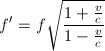, where f is the source frequency, f' is the observed frequency, v is the relative velocity between source and observer, and c is the speed of light.

When the source and observer are moving closer together, v is positive, so the observed frequency is greater than the source frequency. Greater frequency also implies shorter wavelength, so visible light is shifted towards the blue end of the spectrum.

### Example Question #2 : Doppler Effect

A sound source with a frequency of 790Hz moves away from a stationary observer at a rate of 15m/s. What frequency does the observer hear?

The speed of sound is 340m/s.

775Hz

757Hz

655Hz

826Hz

757Hz

Explanation:

In this scenario the Doppler effect is described by the following equation.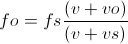Using the values from the problem, we know that vo is zero and vf is 15m/s. v is 340m/s and fs is 790Hz.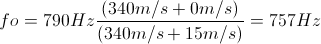### Example Question #3 : Doppler Effect

A fire truck emits an 880Hz siren. As the truck approaches an obeserver on the sidewalk, he perceives the pitch to be 950Hz. Approximately what pitch does he hear after the truck passes and is moving away? Assume the truck's velocity remains constant, and that the velocity of sound in air is 340m/s.

830Hz

800Hz

840Hz

810Hz

820Hz

820Hz

Explanation:

The equation for Doppler effect is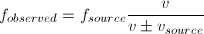, where the + sign applies when the source and observer are moving farther apart, and the - sign applies when they are moving closer together. In these equations, v is the speed of sound, 340m/s,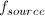is the frequency of sound emitted by the source,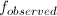is the freqency perceived by the observer, and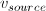is the relative velocity between the source and observer.

We can apply this equation to the first part of the motion, as the truck moves closer to the observer, to solve for the velocity of the truck.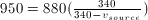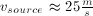Now we can plug this velocity into the equation again for when the truck moves farther away from the observer and solve for.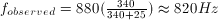### Example Question #4 : Doppler Effect

At a local concert, a speaker is set up to produce low-pitched base sounds with a frequency range of 20Hz to 200Hz, which can be modeled as  sine waves. In a simplified model, the sound waves the speaker produces can be modeled as a cylindrical pipe with one end closed that travel through the air at a velocity of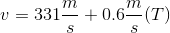, where T is the temperature in °C.

As a person walks towards the speaker, the frequency he or she hears __________.

remains the same

decreases

increases or decreases, depending on temperature

increases

increases

Explanation:

This question is asking us how the frequency changes when one object moves directly towards another; thus, this is a Doppler effect problem. Remembering back to our Doppler effect formula, we know that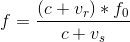, where f is the frequency heard by the recipient (the person at the concert), vr is the velocity of the receiver, vs is the velocity of the source, and f0 is the original frequency.

In our case, the speaker is not moving, so vs is zero. vr is positive when the person is walking towards the speaker, so the frequency heard will be higher than the original frequency.

### Example Question #5 : Doppler Effect

At a local concert, a speaker is set up to produce low-pitched base sounds with a frequency range of 20Hz to 200Hz, which can be modeled as  sine waves. In a simplified model, the sound waves the speaker produces can be modeled as a cylindrical pipe with one end closed that travel through the air at a velocity of, where T is the temperature in °C.

A person runs away from the speaker at 3m/s, while it creates a 200Hz sound wave. What frequency does he or she hear?

Use 340m/s for the speed of sound.

220.9Hz

190.7Hz

198.2Hz

201.3Hz

198.2Hz

Explanation:

This question is asking us how the frequency changes when one object moves directly away from another; thus, this is a Doppler effect problem. Remembering back to our Doppler effect formula, we know that, where f is the frequency heard by the recipient (the person at the concert), vr is the velocity of the receiver, vs is the velocity of the source, and f0 is the original frequency.

In our case, the speaker is not moving, so vs is zero. vr is negative when the person is walking towards the speaker, so the frequency heard will be lower than the original frequency. We can calculate the heard frequency using our equation.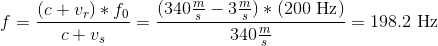### Example Question #6 : Doppler Effect

The source of a sound moves away from the listener. The listener has the impression that the source is __________.

lower in pitch

higher in amplitude

higher in tone

lower in velocity

lower in pitch

Explanation:

The formula for the Doppler effect is: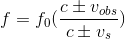Only frequency of the sound is affected by the Doppler effect; velocity and amplitude remain unchanged. When the source is moving away from the observer the velocity of the source is added to the speed of light.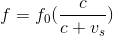This increases the value of the denominator, decreasing the value of the observed frequency. Frequency corresponds to pitch or tone; a lower observed frequency will result in a lower observed pitch.

### Example Question #7 : Doppler Effect

You are jogging on the sidewalk at a rate of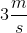. A police car behind you is patrolling at a rate of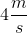when it turns on its siren. If the siren has a frequency of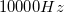, is the frequency you perceive higher or lower than the frequency emitted?

Lower because the overall distance between you and the siren is increasing

Higher because the siren is traveling the same direction as you

Neither higher nor lower because the doppler effect only affects sounds at lower frequencies

Lower because you are running slower than the police car

Higher because the overall distance between you and the siren is decreasing

Higher because the overall distance between you and the siren is decreasing

Explanation:

The doppler effect states that if two objects are moving closer together, perceived frequencies for emitted waves will be higher. If you are jogging away from the car at, but the car is traveling at, the overall distance between you and the siren is decreasing. You will hear a higher frequency than what the siren is emitting.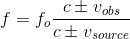The numerator is subtracted when the observer moves away from the source. The denominator is subtracted when the source moves toward the observer. In this problem both terms would be subtracted, but the denominator would be decreased by more than the numerator. This would result in a fraction greater than one, and an overall increase in the final frequency.

### Example Question #8 : Doppler Effect

A star emits visible color at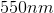. If the star is moving away from a stationary observer, which of the following cannot be the wavelength observed?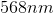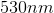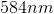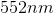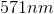Explanation:

The Doppler effect accounts for observed frequency versus actual frequency emitted by a sound or light source. The equation for the Doppler effect is:The numerator terms are summed when the observer moves toward the source, and the denominator terms are summed when the source moves away from the observer. In this question, we know that the source moves away from the observer; therefore, the observed frequency will be less than the actual frequency because the denominator in the equation will increase.

The question, however, asks about wavelength. If the frequency decreases, then the wavelength must increase according to the equation: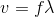Velocity will remain constant (the speed of light). Any change in frequency will cause a change in wavelength via their direct relationship in the given equation.

### Example Question #9 : Doppler Effect

Two cars approach each other at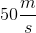when one car starts to beep its horn at a frequency of 475Hz. What is the wavelength of the horn as heard by the other driver?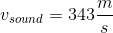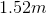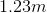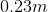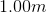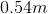Explanation:

The Doppler equation is: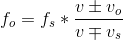.

Because the cars are approaching each other, the frquency heard will be increased. This fundamental knowledge allows you to determine the signs of the equation. The top of the fraction will be addition and the bottom will be subtraction to make a coefficient greater than 1.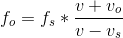Use the given values to solve: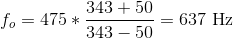Now that we know the frequency, we can solve for the wavelength: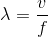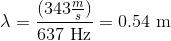### Example Question #10 : Doppler Effect

A fire truck emitting a siren at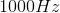moves at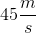towards a jogger. The jogger is moving at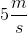towards the fire truck. Take the speed of sound to be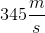.

At what frequency does the jogger perceive the siren?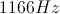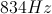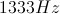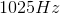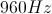Explanation:

In order to solve this problem we must know how to utilize the Doppler formula.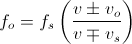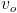is the velocity of the observer and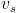is the velocity of the source. Notice that the frequency must increase as the observer and source move closer, and therefore the plus sign is used in the numerator and the minus sign is used in the denominator. Had the jogger been moving away from the fire truck, the subtraction function would be used in both the top and bottom.

In this case we see that the source is the fire truck, moving at, and the observer is the jogger, moving at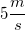. By plugging these numbers into the formula andfor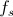, we find the perceived frequency or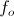to be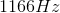.